International
Tables for
Crystallography
Volume C
Mathematical, physical and chemical tables
Edited by E. Prince

International Tables for Crystallography (2006). Vol. C, ch. 9.8, pp. 936-937

Section 9.8.3.6. Ambiguities in the notation

T. Janssen,a A. Janner,a A. Looijenga-Vosb and P. M. de Wolffc

aInstitute for Theoretical Physics, University of Nijmegen, Toernooiveld, NL-6525 ED Nijmegen, The Netherlands,bRoland Holstlaan 908, NL-2624 JK Delft, The Netherlands, and cMeermanstraat 126, 2614 AM, Delft, The Netherlands

9.8.3.6. Ambiguities in the notation

| top | pdf |

The invariant part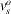of the translation part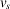of a (3 + 1)-dimensional superspace-group element is uniquely determined by (9.8.3.5). This does not imply that for each element of the point group there is a translation for which the invariant part is unique up to lattice vectors. The reason is that, for a given element R of the point group and given origin,may be changed when R is combined with a three-dimensional lattice translation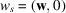. This situation is well known in ordinary three-dimensional crystallography. For example, the twofold rotation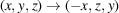in the space grouphas according to Volume A of International Tables for Crystallographya translation part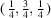. Its invariant part is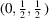. However, when the translation part is equivalently taken as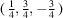, the invariant part vanishes. Therefore, in the symbol for that space group, the corresponding generator is given as the rotation 2' and not as the screw axis'.

The same situation may occur in 3 + 1 dimensions. This can be seen very clearly from the definition of τ [equation (9.8.3.8)]. Since v is only determined modulo a lattice vector, one may add to it a lattice vector that has a non-vanishing product with qr. This results in a change for τ. For example, the (3 + 1)-dimensional space group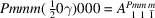has a mirror perpendicular to the a axis with associated value τ = 0. The parallel mirror at a distance a/2 has v = a and consequently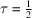. Hence, the symbols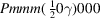and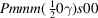indicate the same group. This non-uniqueness in the symbol, however, does not have serious practical consequences.

Another source of ambiguity is the fact that the assignment of a satellite to a main reflection is not unique. For example, the reflection conditions for the group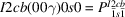are h + k + l = even because of the centring and l + m = even and h + m = even for h0lm because of the two glide planes perpendicular to the b axis. When one takes for the modulation vector q = γ′c* = (1 − γ)c*, the new indices are h, k, l′, and m′ with l′ = l + m and m′ = −m. Then the reflection conditions become l′ = even and h + m = even for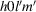. The first of these conditions implies the symbol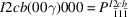for the group considered. This, however, is the symbol for the nonequivalent group with condition h = even for h0lm. This difficulty may be avoided by sometimes using a non-standard setting of the three-dimensional space group (see Yamamoto et al., 1985). In this case, the setting I2ab instead of I2cb avoids the problem.

References

International Tables for Crystallography (2005). Vol. A, edited by Th. Hahn, fifth ed. Heidelberg: Springer.
Yamamoto, A., Janssen, T., Janner, A. & de Wolff, P. M. (1985). A note on the superspace groups for one-dimensionally modulated structures. Acta Cryst. A41, 528–530.